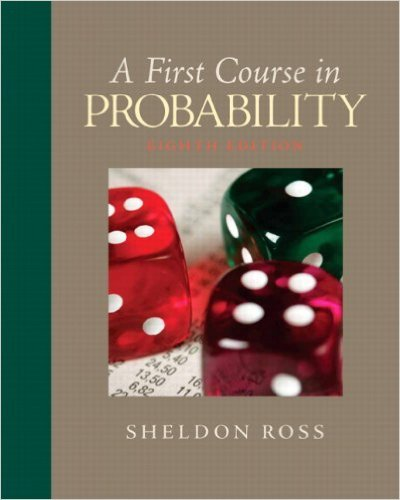×
×

# Consider the following technique for shuffling adeck of nISBN: 9780136033134 163

## Solution for problem 22 Chapter 2

First Course in Probability | 8th Edition

• Textbook Solutions
• 2901 Step-by-step solutions solved by professors and subject experts
• Get 24/7 help from StudySoup virtual teaching assistantsFirst Course in Probability | 8th Edition

4 5 1 383 Reviews
13
4
Problem 22

Consider the following technique for shuffling adeck of n cards: For any initial ordering of thecards, go through the deck one card at a time andat each card, flip a fair coin. If the coin comesup heads, then leave the card where it is; if thecoin comes up tails, then move that card to theend of the deck. After the coin has been flipped ntimes, say that one round has been completed. Forinstance, if n = 4 and the initial ordering is 1, 2, 3,4, then if the successive flips result in the outcomeh, t, t, h, then the ordering at the end of the roundis 1, 4, 2, 3. Assuming that all possible outcomes ofthe sequence of n coin flips are equally likely, whatis the probability that the ordering after one roundis the same as the initial ordering?

Step-by-Step Solution:
Step 1 of 3
Step 2 of 3

Step 3 of 3

##### ISBN: 9780136033134

Unlock Textbook Solution